﻿ 一类随机金融系统的平衡控制和随机抑制

# 一类随机金融系统的平衡控制和随机抑制Equilibrium Control and Stochastic Suppression of a Class of Stochastic Financial Systems

Abstract: A stochastic financial system is constructed and its dynamic properties are analyzed. Based on the stochastic sensitivity function technique, a stochastic sensitivity matrix is synthesized. In view of the stochastic attractor generated by the stochastic system deviating from the determinate at-tractor, a feedback-regulated equilibrium point of the partially stable stochastic system is designed, and a new regulation is made around the equilibrium point. It can reduce the sensitivity of stochastic systems and suppress random oscillations.

1. 引言

2. 一类确定性金融系统的参数分析

2.1. 一类金融系统的改进

$\left\{\begin{array}{l}\stackrel{˙}{x}=z+\left(y-a\right)x\hfill \\ \stackrel{˙}{y}=1-by-{x}^{2}\hfill \\ \stackrel{˙}{z}=-x-cz\hfill \end{array}$ (1)

$\left\{\begin{array}{l}\stackrel{˙}{x}=rz+\left(y-a\right)x+kx\hfill \\ \stackrel{˙}{y}=1-by-{x}^{2}\hfill \\ \stackrel{˙}{z}=-x-cz\hfill \end{array}$ (2)

2.2. 确定性系统局部稳定性与分岔

${E}_{1}=\left(0,\frac{1}{b},0\right)$

${E}_{2}=\left(\sqrt{1-\frac{br}{c}-ab+kb},\frac{r}{c}+a-k,-\frac{1}{c}\sqrt{1-\frac{br}{c}-ab+kb}\right)$

${E}_{3}=\left(-\sqrt{1-\frac{br}{c}-ab+kb},\frac{r}{c}+a-k,\frac{1}{c}\sqrt{1-\frac{br}{c}-ab+kb}\right)$

2.2.1. 平衡点E1的pitchfork分岔与Hopf分岔

${F}_{1}\left(\lambda \right)=\left(\lambda +b\right)\left[\left(\frac{1}{b}-a+k-\lambda \right)\left(c+\lambda \right)-r\right]=0$

2.2.2. 平衡点E2和E3的Hopf分岔

${F}_{2,3}\left(\lambda \right)={\lambda }^{3}+p{\lambda }^{2}+q\lambda +s=0$

$q=2kb+bc+2-\frac{3br}{c}-2ab$

$s=2kbc+2c-2br-2abc$

$B=\frac{2abc-3{b}^{2}c-2c-2kbc}{3b}$

$C=\frac{2b{c}^{2}+{b}^{2}{c}^{3}+b{c}^{4}-2a{b}^{2}{c}^{2}+2k{b}^{2}{c}^{2}}{3b}$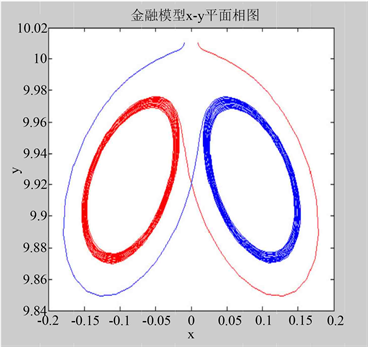$r=0.978$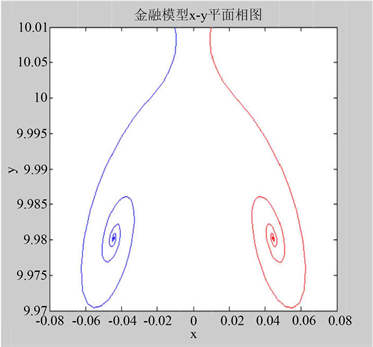$r=0.9132$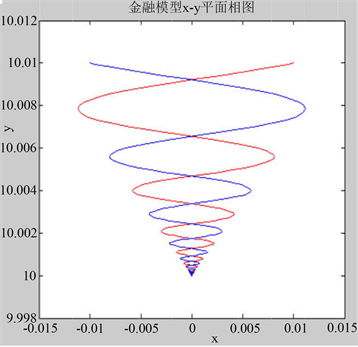$r=0.8$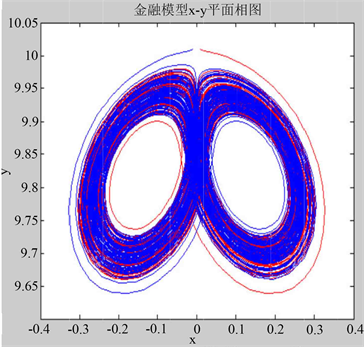$r=2$

Figure 1. Deterministic system attractor

3. 随机平衡点的灵敏度

$\left\{\begin{array}{l}\stackrel{˙}{x}=rz+\left(y-a\right)x+kx+\epsilon {\stackrel{˙}{w}}_{1}\hfill \\ \stackrel{˙}{y}=1-by-{x}^{2}+\epsilon {\stackrel{˙}{w}}_{2}\hfill \\ \stackrel{˙}{z}=-x-cz+\epsilon {\stackrel{˙}{w}}_{3}\hfill \end{array}$ (3)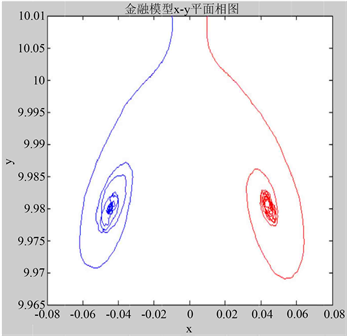$r=0.978$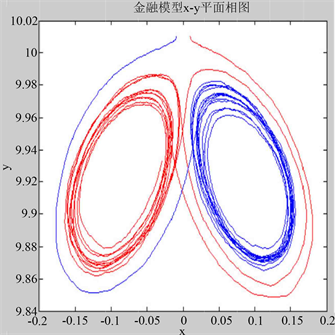$r=0.9132$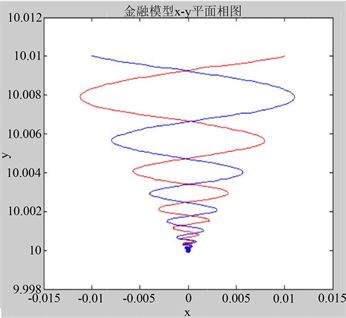$r=0.8$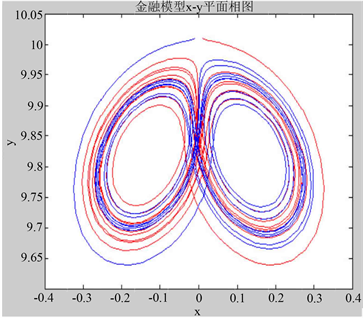$r=2$

Figure 2. Attractor of stochastic financial system ( $\epsilon =0.0001$ )

$\stackrel{˙}{x}=f\left(x\right)dt+\epsilon \sigma \stackrel{˙}{w}$ (4)

$x$ 是一个3维向量， $w\left(t\right)$ 是一个3维维纳过程， $\epsilon$ 是强度参数。

$\sigma =\left[\begin{array}{ccc}1& 0& 0\\ 0& 1& 0\\ 0& 0& 1\end{array}\right]$

$\epsilon =0$ ，假设系统(4)有指数稳定的平衡点，这样随机系统(4)的随机轨迹就会离开平衡位置并在其周围形成带有平稳概率分布 ${\rho }_{t}\left(x,\epsilon \right)$ 的随机吸引子(如图2)， ${\rho }_{t}\left(x,\epsilon \right)$ 是Fokker-Planck方程的解，但是即使在二维的情况下，这个方程也很难获得解析解。对于小噪声，我们可以得到 ${\rho }_{t}\left(x,\epsilon \right)$ 的近似 ${\rho }_{t}^{*}\left(x,\epsilon \right)$ ，基于拟势函数 。

$v\left(x\right)=-\underset{\epsilon \to 0}{\mathrm{lim}}{\epsilon }^{2}\mathrm{log}\rho \left(x,\epsilon \right)$

${\rho }_{t}\left(x,\epsilon \right)\approx {\rho }_{t}^{\ast }\left(x,\epsilon \right)=N\ast \mathrm{exp}\left(-\frac{{\left(x-\stackrel{¯}{x}\right)}^{T}{W}^{-1}\left(x-\stackrel{¯}{x}\right)}{2{\epsilon }^{2}}\right)$

$AW+W{A}^{T}+S=0$ (5)

$W=\left[\begin{array}{ccc}445.4985& -165.9164& -393.8766\\ -165.9164& 153.3293& 166.0713\\ -393.8766& 166.0713& 354.9389\end{array}\right]$

4. 随机系统的稳定控制

$\stackrel{˙}{x}=f\left(x,u\right)+\epsilon \sigma \stackrel{˙}{w}$ (6)

$u={k}_{1}\left({x}_{1}-\stackrel{¯}{x}\right)+{k}_{2}\left({y}_{\text{1}}-\stackrel{¯}{y}\right)+{k}_{3}\left({z}_{\text{1}}-\stackrel{¯}{z}\right)$

$dz=Az+\epsilon \sigma \stackrel{˙}{w}$

$dz=\left(A+BK\right)zdt+\epsilon \sigma \stackrel{˙}{w}$

$\left(A+BK\right)W+W{\left(A+BK\right)}^{T}+S=0$ (7)

$M$ 是随机灵敏度矩阵的集合，其中元素满足对称和正定性。

$\Phi =\left\{K|\mathrm{Re}{\lambda }_{i}\left(A+BK\right)<1\right\},{\lambda }_{i}\left(A\right)$ 是矩阵A的特征值。

${P}_{2}H\left(\left(W\right){P}_{2}=0$ (8)

$K=\stackrel{¯}{K}+C,\stackrel{¯}{K}={B}^{+}H\left(W\right)\left(\frac{1}{2}{P}_{1}-I\right){W}^{-1}$ (9)

C是满足 $BCW+W{C}^{T}{B}^{T}=0$ 任意的 $\text{1}×\text{3}$ 矩阵。

5. 随机受控金融系统的数值模拟

$\left\{\begin{array}{l}\stackrel{˙}{x}=rz+\left(y-a\right)x+kx+u+\epsilon {\stackrel{˙}{w}}_{1}\hfill \\ \stackrel{˙}{y}=1-by-{x}^{2}+\epsilon {\stackrel{˙}{w}}_{2}\hfill \\ \stackrel{˙}{z}=-x-cz+\epsilon {\stackrel{˙}{w}}_{3}\hfill \end{array}$ (10)

$E\left({w}_{i}\left(t\right)-{w}_{i}\left(s\right)\right)=0,E{\left({w}_{i}\left(t\right)-{w}_{i}\left(s\right)\right)}^{2}=|t-s|$$u$ 是控制部分。由图3可以看出在 $\left[0.5,0.55\right]$$\left[0.9,0.99\right]$ 之间，平衡点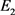的随机灵敏度非常大。

$A=\left[\begin{array}{ccc}\stackrel{¯}{y}-a+k& \stackrel{¯}{x}& r\\ -2\stackrel{¯}{x}& -b& 0\\ -1& 0& -c\end{array}\right],B=\left[\begin{array}{c}1\\ 0\\ 0\end{array}\right],$

$K=\left[\begin{array}{ccc}{k}_{1}& {k}_{2}& {k}_{3}\end{array}\right],S=\left[\begin{array}{ccc}1& 0& 0\\ 0& 1& 0\\ 0& 0& 1\end{array}\right],$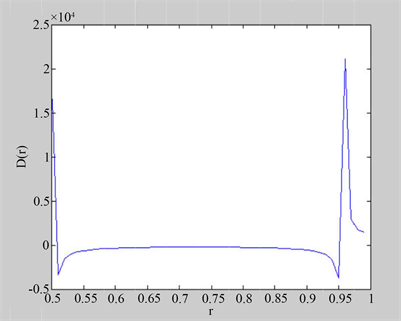Figure 3. Stochastic sensitivity of equilibrium point ${E}_{2}$ of uncontrolled financial system

${P}_{1}=\left[\begin{array}{ccc}1& 0& 0\\ 0& 0& 0\\ 0& 0& 0\end{array}\right],{P}_{2}=\left[\begin{array}{ccc}0& 0& 0\\ 0& 1& 0\\ 0& 0& 1\end{array}\right],$

$W=\left[\begin{array}{ccc}{w}_{11}& {w}_{12}& {w}_{13}\\ {w}_{12}& {w}_{22}& {w}_{23}\\ {w}_{13}& {w}_{23}& {w}_{33}\end{array}\right].$

$\left\{\begin{array}{l}-4\stackrel{¯}{x}{w}_{12}-2b{w}_{22}+1=0\hfill \\ -2{w}_{13}-2c{w}_{33}+1=0\hfill \\ -2\stackrel{¯}{x}{w}_{13}-{w}_{12}-\left(b+c\right){w}_{23}=0\hfill \end{array}$

${w}_{12}=-0.4\stackrel{¯}{x},{w}_{22}=\frac{1+1.6{\stackrel{¯}{x}}^{2}}{2b},{w}_{33}=\frac{3}{10c}$

$W=\left[\begin{array}{ccc}1& -0.4\stackrel{¯}{x}& 0.2\\ -0.4\stackrel{¯}{x}& \frac{1+1.6{\stackrel{¯}{x}}^{2}}{2b}& 0\\ 0.2& 0& \frac{3}{10c}\end{array}\right]$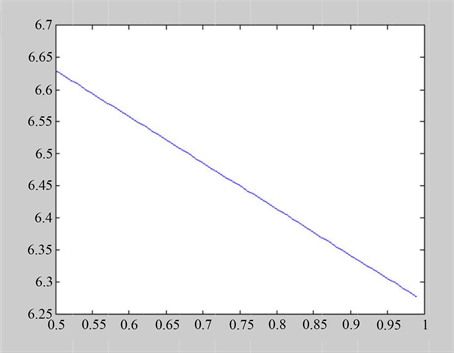Figure 4. Stochastic sensitivity of equilibrium point ${E}_{2}$ of controlled financial system

$r=0.9132$ 时， $K=\left[-2.3746,2.2492,4.7637\right]$

$r=0.978$ 时， $K=\left[-2.5057,1.1175,4.7528\right]$

 时， $K=\left[-2.1411,3.4952,4.7794\right]$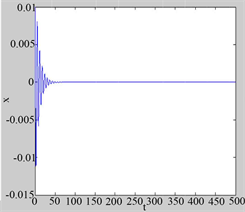$r=0.8$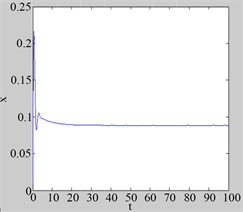$r=0.8$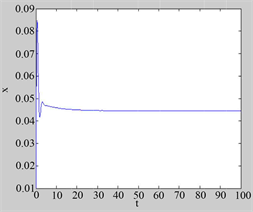$r=0.978$

Figure 5. Stability of random oscillation in x-coordinate of random trajectory

6. 结论

1) 不加控制项的随机系统的随机轨迹会发散在平衡位置周围，加入控制项后，可以将随机轨迹稳定在平衡位置的小邻域内。

2) 加入控制项后可以实现随机振荡的稳定，并且其稳定性的实现是由于受控系统平衡位置的随机灵敏度的降低。

 Jungeilges, J. and Ryazanova, T. (2017) Noise-Induced Transitions in a Stochastic Goodwin-Type Business Cycle Model. Structural Change and Economic Dynamics, 40, 103-115.
https://doi.org/10.1016/j.strueco.2017.01.003

 Mil’shtein, G.N. and Ryashko, L.B. A First Approximation of the Quasipotential in Problems of the Stability of Systems with Random Non-Degenerate Perturba-tions.

 Bashkirtseva, I. and Ryashko, L. Stochastic Sensitivity Analysis of Noise-Induced Intermittency and Tran-sition to Chaos in One-Dimensional Discrete-Time.

 Bashkirtseva, I. and Ryashko, L. Stochastic Sensitivity Analysis of Noise-Induced Order-Chaos Transitions in Discrete-Time Systems with Tangent and Cri-sis.

 Bashkirtseva, I., Ryazanova, T. and Ryashko, L. (2015) Analysis of Dynamic Regimes in Stochastically Forced Kaldor Model. Chaos, Solitons and Fractals, 79, 96-104.
https://doi.org/10.1016/j.chaos.2015.02.019

 Bashkirtseva, I., Ryashko, L. and Sysolyatina, A. (2016) Analysis of Stochastic Effects in Kaldor-Type Business Cycle Discrete Model. Communications in Nonlinear Science and Numerical Simulation, 36, 446-456.
https://doi.org/10.1016/j.cnsns.2015.12.020

 Bashkirtseva, I.A. and Ryashko, L.B. (2000) Sensitivity Analysis of the Stochastically and Periodically Forced Brusselator. Physica A, 278, 126-139.
https://doi.org/10.1016/S0378-4371(99)00453-7

 Bashkirtseva, I. and Ryashko, L. (2002) Sensitivity Analysis of Stochastically Forced Lorenz Model Cycles under Period-Doubling Bifurcations. Dynamic Systems and Applica-tions.

 Bashkirtseva, I., Chen, G.R. and Ryashko, L. (2012) Stochastic Equilibria Control and Chaos Suppression for 3D Systems via Stochastic Sensitivity Synthesis. Communications in Nonlinear Science and Numerical Simulation, 17, 3381-3389.
https://doi.org/10.1016/j.cnsns.2011.12.004

 Bashkirtseva, I.A. and Ryashko, L.B. (2004) Stochastic Sensitivity of 3D-Cycles. Mathematics and Computers in Simulation, 66, 55-67.
https://doi.org/10.1016/j.matcom.2004.02.021

 杨明证. 一类改进的混沌金融系统的混沌同步研究[D]: [硕士学位论文]. 镇江: 江苏大学, 2010.

 Dembo, M. and Zeitouni, O. (1995) Large Deviations Techniques and Applications. Jones and Bartlett, Boston.

 Mil’shtein, G.N. and Ryashko, L.B. (1995) A First Approximation of the Quasipotential in Problems of the Stability of Systems with Random Non-Degenerate Perturbations. Journal of Applied Mathematics and Mechanics, 59, 47-56.
https://doi.org/10.1016/0021-8928(95)00006-B

Top